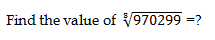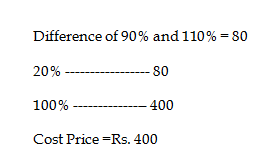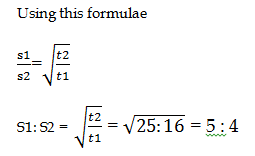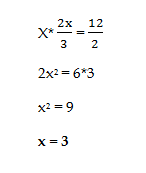# RRB ALP 2018 Practice Test Papers | Arithmetic Questions (Day-67)

Dear Aspirants, Here we have given the Important RRB ALP & Technicians Exam 2018 Practice Test Papers. Candidates those who are preparing for RRB ALP 2018 can practice these Arithmetic Questions to get more confidence to Crack RRB 2018 Examination.

[WpProQuiz 2933]

Click “Start Quiz” to attend these Questions and view Solutions

1) What is the sum of the measures of the interior angles of a hexagon?

a) 540°

b) 720°

c) 810°

d) 108°

2) Present Age of Aditi and Buvi is 4: 1. Difference of their ages is 15 years. What is the sum of their ages?

a) 25

b) 15

c) 20

d) 10

3) Poonam borrowed a sum of amount with the interest of 10% of Simple Interest per annum. He returned 600 as interest after 5 years. Find the principal.

a) 300

b) 600

c) 1200

d) 2400

4) Three pipes P, Q and R are filled a tank in 25 mins, 150 mins and 50mins respectively. All three pipes are opened simultaneously when will the tank fill?

a) 12 mins

b) 15 mins

c) 12 ½ mins

d) 16 mins

5)a) 96

b) 97

c) 99

d) 98

6) 98% of 500 + 142361 X 19

a) 345

b) 325

c) 375

d) 350

7) Pravin earns 25 % more than Pritvi. Then how much Pritvi earn less percentage than Pravin?

a) 20 %

b) 25 %

c) 33.33 %

d) 30 %

8) A person sold an article with the loss of 10%. If he sold it Rs.80 more he gains 10% profit. What is the cost price of the article?

a) 100

b) 200

c) 300

d) 400

9) Two trains, one from Chennai to Delhi and the other from Delhi to Chennai start simultaneously. After they meet, the trains reach their destinations after 16 hours and 25 hours respectively. The ratio of their speeds per hour is?

a) 4: 5

b) 3: 4

c) 5: 4

d) 4: 3

10) A number is multiplied with its 2/3rd of the same number gives half of 12. Find the number.

a) 13

b) 5

c) 3

d) 2

The sum of the measures of the interior angles of a polygon with n sides is 180. (n – 2) A hexagon has six sides. Therefore, substitute 6 for n into the formula and calculate.

180(6 – 2) = 180(4) = 720

Therefore, the sum of the measures is 720°.

4x – x = 15

3x = 15

X = 5

4x = 4×5 = 20; x = 5

4x + x = 5x = 25

S.I = PNR/100

600 = P×10/100× 5

(600×100)/5×10 = P

P = 1200

P – 1/25 mins

Q – 1/150 mins

R – 1/ 75 mins

P+Q+R = 1/25 + 1/150 + 1/50

PQR = (6 + 1+ 3)/ 150 = 10 / 150

Pipes PQR  fill the tank in 15 mins

(99)3 = 970299

490 + 196 – 19 ×19 = 686 – 361 = 325

Pravin             :          Pritvi

125                  :           100

(25/125) × 100 = 20%